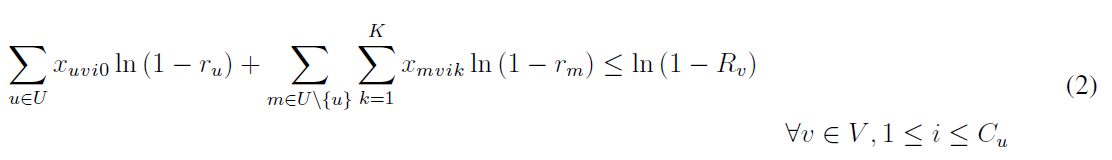•Gurobi Staff

Hi Hernani,

What is the exact error you are getting? Could you post the code you have used so far to implement this constraint?

Best regards,
Jaromił

•Hi Jaromil,

My apologize I though I copy the code to the post. Here is how I coded the constraint,

model.addConstrs(    quicksum(        x[u, v, i, 0] * math.log(1 - r_u[u]) + x[m, v, i, k] * math.log(1 - r_u[m])        for m in U        for k in range(K)        if u != m    )    <= math.log(1 - R_v[v])    for v in V    for i in range(C_u[u]))
Regards,
Hernani
•Gurobi Staff

Hi Hernani,

I suppose $$r_u$$ refers to a Gurobi variable. In this case, you cannot simply apply the logarithm to this variable and then use the result in a constraint. You first need to add auxiliary variables to capture these logs and then use those variables in the final constraint.

I hope that helps.

Cheers,
Matthias

•Hi Matthias,

Sorry I did not make it clear but the decision variables are x_uvik, r_u[u] and r_u[m] and R_v[v] are constants.

Regards,

Hernani

•Dear all,

I have this constraint (2) where x_uvik is binary decision variable and r_u, r_m and R_v are parameters to the problem.I coded the problem as follow: But when I run my program with this constraint I get the model is unfeasible unable to retrieve attribute X. Could you please point me the right direction to solve this.

for v in V:
constraint = LinExpr()
constraint1 = LinExpr()
for u in U:
for i in range(C_u[u]):
for m in U:
for j in range(C_u[m]):
if (u!=m):
for k in range(K):
model.addConstr( constraint + constraint1 <=math.log(1-R_v[v]))
Regards,
Hernani
•Gurobi Staff

Hi Hernani,

You could try to solve the IIS of this model to figure out what might be the cause of the infeasibility:
Model.computeIIS() (gurobi.com)

Cheers,
Matthias

•Hi Matthias,

Thank you for quick response, regarding to the code of the constraint for your experience it seems correct or there is any suggestion you would like to share.

Regards,

Hernani

•The mathematical description seems to encode $$|V|*\sum_u C_u$$ constraints, but it looks like you are only creating $$|V|$$ constraints. Could that be the problem?